### 2.2.2.2 Diffusion Current

The component of the current which is caused by the thermal motion of the carriers is called diffusion current. It is driven by a gradient in the carrier concentration. The law of diffusion which originally stems from the theory of dilute gases reads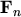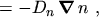(2.29)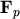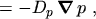(2.30)

where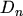and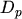are the diffusion coefficients for electrons and holes, respectively,andare the respective particle flux densities, which have to be multiplied by the charge of the particle to get the electrical current density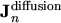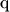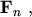(2.31)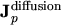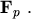(2.32)

For conditions close to thermal equilibrium and for non-degenerate carrier systems (BOLTZMANN statistics), the diffusion coefficients are related to the mobilities by the EINSTEIN relation(2.33)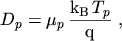(2.34)

where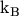is BOLTZMANN's constant.

Superposition of the current components yields the drift-diffusion current relations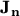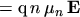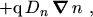(2.35)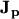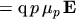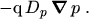(2.36)

M. Gritsch: Numerical Modeling of Silicon-on-Insulator MOSFETs PDF